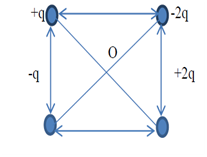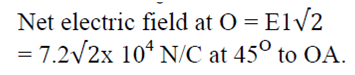## Sunday, 20 March 2016

### WORKSHET#3 ELECTRIC FIELD CBSE CLASS 12 PHYSICS QUESTIONS WITH KEY

1.Two point charges, q =8×10-8 C and Q= -2×10-8 C are separated by a distance of 10 cm in air. What is the net electric field at the mid- point between the charges.

Using the formulae=F/Q =3.6x 105

2. Two point charges 4 Q, Q are separated by 1 m in air. At what point on the line joining the charges is the electric field intensity zero?  Q9
x=2/3 m
3 .Two point charges of 16μC and -9μC are placed 8cm apart in air. Determine the position of the point at which resultant electric field is zero.
4 .Eight identical point charges of q Coulomb each are placed at corners of a cube of each side 0.1m .Calculate electric field at the center of the cube. Calculate the field at the center when one of the corner charges is removed.
i) zero ii) E = (1.2 x 1012)q N/C

5. What are the magnitude and direction of electric field at the center of the square in fig if q=1.0 x 10 -8C and a = 5cm?6. A charge of 4 x 10 -9 C is distributed uniformly over the circumference of a conducting ring of radius 0.3m. Calculate the field intensity at a point on the axis of the ring at 0.4m from its centre, also at the center.

At the center of ring, r = 0,
So E = 0
7. Charge q1=+6.0nC is on y axis at y=+3 cm and charge q2 = -6nCis on y axis at y=-3cm .Calculate force on test charge q0= 2 nC placed on x axis at x=4cm.

-51.8 j μN

8.ABC is an equilateral triangle of each side 5 cm. two charges of ±50/3 x 10-3 μC are placed at A and B respectively. Calculate magnitude and direction of resultant intensity at C.

6 x 104 N/C parallel to AB from + charge to – charge.

### WORKSHEET#2 ELCTRIC FLUX CBSE CLASS12 PHYSICS QUESTIONS WITH KEY

1. If the radius of the Gaussian surface enclosing a charge is halved, how does the electric flux through the Gaussian surface change?
No change
2. A charge Q micro coulomb is placed at the centre of a cube. What would be the flux through one face?
Q/6ε0

3. If the E=8i+4j+3k NC-1, Calculate the electric flux through a surface of area 100 m2 lying in x-y plane
ɸ= EScosθ =30 Nm2c-1 ii.15 Nm2C-1
300 Nm2C-1

4.The electric field in a certain region of space is (5i+4j-4k) x 105 N/C. Calculate the electric flux due to this field over an area of (2i-j) x 10-2 m2.

ɸ=E.S = 6 x 103 Nm2C-1

5. A sphere of radius 0.03m has a point charge of q = 7.6μC is located at its center. Find the electric flux
through it.

Φ=q/ɛ0 = 8.6 x 105Nm2C-1

6. A point charge of 17.7μC is located at the centre of a cube of side 0.03m. Find the electric flux through each face of the cube.

1/6φ=1/6q/ɛ0 = 3.3 x 105Nm2C-1

7. 5,000 lines of force enter a certain volume of space and three thousands lines emerged from it. What is the total charge in Coulomb within the volume?

-1.77 x 10-8 C

8.  A spherical Gaussian surface encloses a charge of 8.85 x 10-8 C
i. Calculate the electric flux passing through the surface
ii. If the radius of the Gaussian surface is doubled, how would the flux change?

i)104 Nm2C-1 ii) 104 Nm2C-1

9.The electric field in a region is given by E=E0x/b i’ find the charge contained in the cubical volume bounded by the surfaces x=0,x=a, y=0,z=0 and z=a. Take E0=5 x 103 Nc-1, a=1cm and b=2cm.
φE=φL

10.An electric field (2i + 3j ) x 103 NC-1 exists in a region. Calculate the electric flux linked with a square of side 0.5m held parallel to a) y-z plane b) x-y plane.

a)24 Nm2 C-1  b) 0.5 Nm2 C-1

### WORKSHEET #1 ELECTRIC CHARGE AND COLLOUMB'S LAW CLASS12 PHYSICS CBSE QUESTIONS WITH KEY

1.An attractive force of 5N is acting between two charges of 2μc and -2μc placed at some distance. If the charges are mutually touched and placed again at the same distance, what will be the new force between them.
zero
2. Force of attraction between two charges at a distance d is F. What distance part should they kept in the medium so that force between them is F/3.
New distance = (3)1/2r
3. The force between two point charges kept at a distance d apart in air is F. If these charges are kept at same distance in water, how does the force between them is affected?
F’=F/K
4 A metal sphere is held fixed on a smooth horizontal insulated plate and another metal sphere is placed at some distance away. If the fixed sphere is given a charge, how will the other sphere react?
When fixed sphere is given a charge it induces opposite charges on the nearer end and same kind of charges on the farther end of the other sphere. Now the net force between the two spheres is attractive in nature because unlike charges on both spheres are closer than the like charges on both spheres. So other sphere will be attracted towards the fixed sphere.
5. Two point charges q1 and q2 are 3 m apart and their combined charge is 20 μc. If one repels the other with a force of 0.075 N, what are two charges?
Q 1= 15 μc and Q2 = 5 μc.
6 A charge P repels charge Q but it attracts charge R. If R repels positively charged Body S, then find nature of charge on Q.
Q is negative
7. The force of attraction between two point charges placed at distance d apart in a medium is F. What should be the distance apart in the same medium so that the force of attraction between them becomes F/4?
New distance=2d
8 Two point charges of 2 μC and 6 μC repel each other with a force of 12 N. If each is now given additional charge of -4 μc, find new force?
F1 = -4 N.

9. Two point charges repel each other with a force of 100 N. One of these charges is increased by 10% and other is reduced by 10%.Find the new force of repulsion between them if distance is kept same.

F’==99N

10. The sum of two point charges is 6µc. They attract each other with a force of 0.9 N. When kept 40 cm apart in vacuum. Find their charges.
q1= 8 X 10 -6 C and q2 = -2 X 10 -6 C

11. Two identical charges Q each, kept at a distance r from each other. A third charge q is placed on the line joining the above two charges such that all the three charges are in equilibrium. What is the magnitude, Sign and position of the charge q?

q =Q ( x = r /2 )